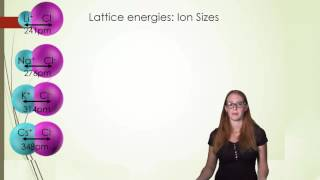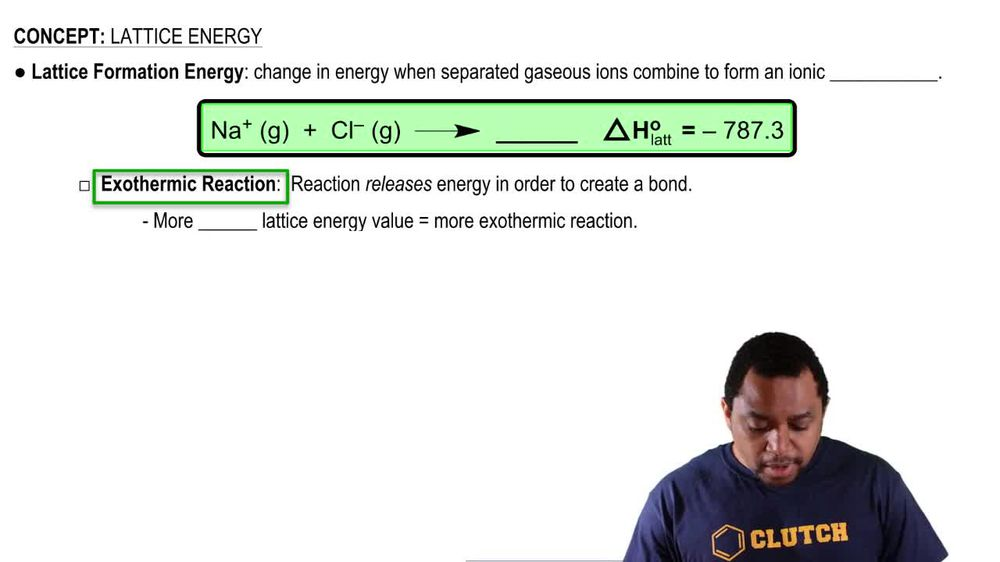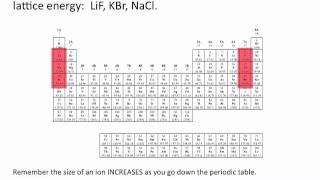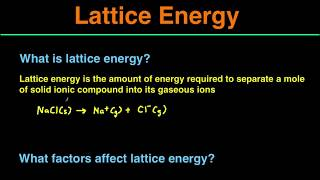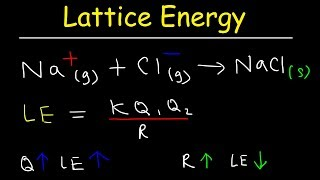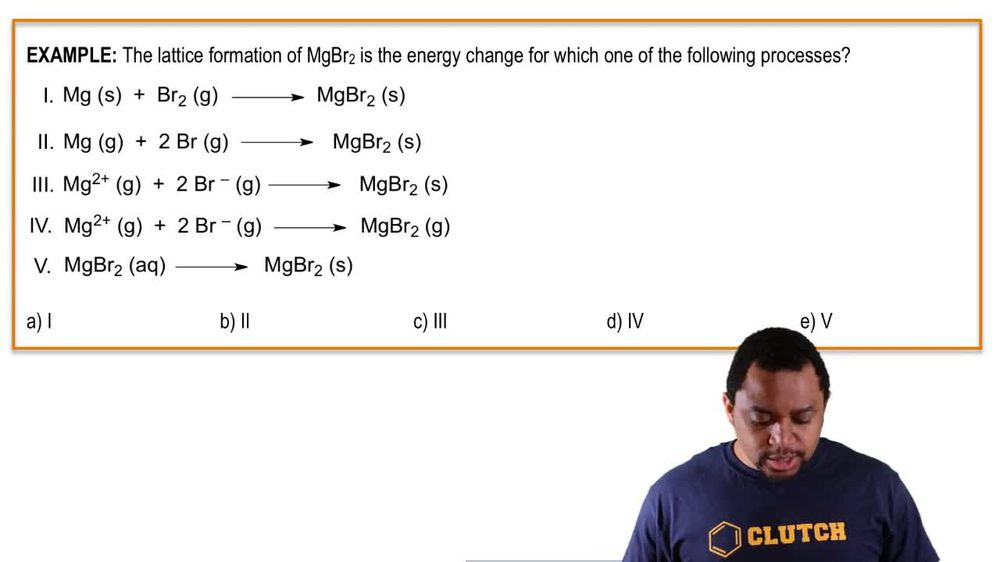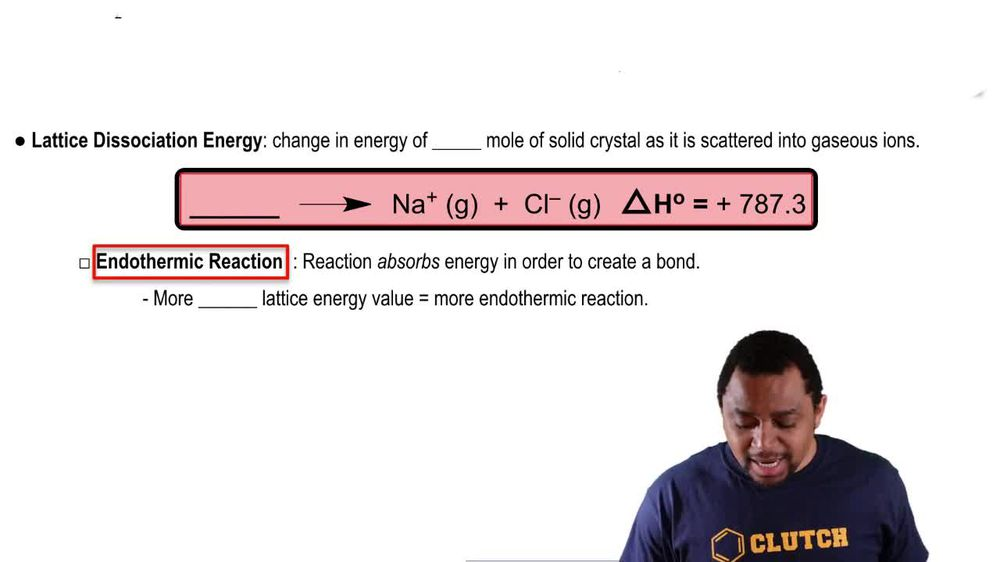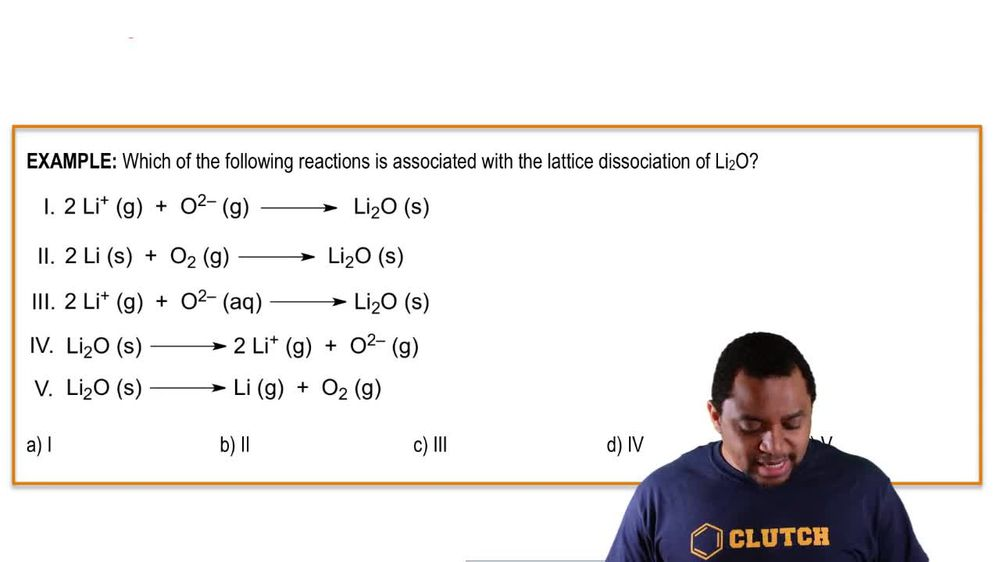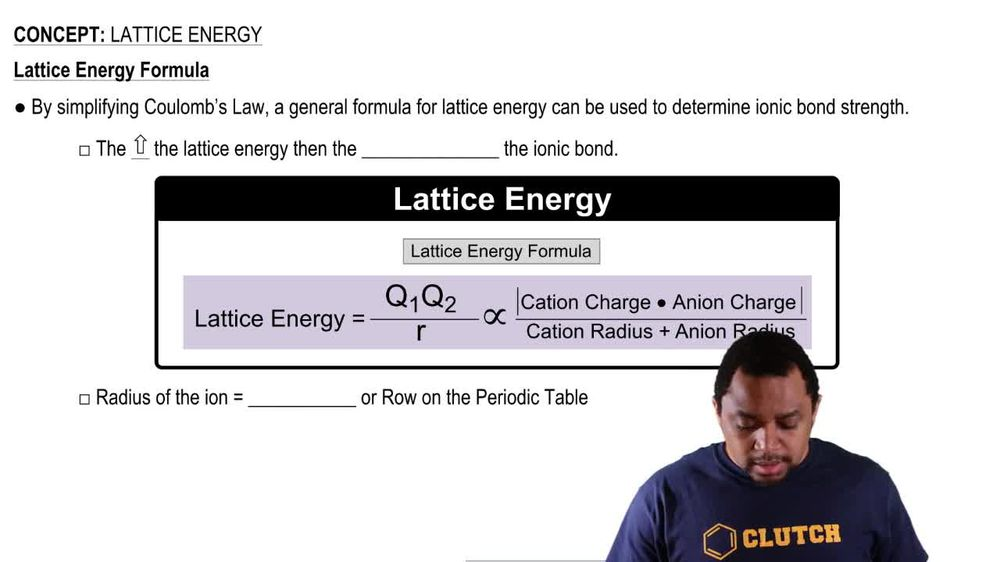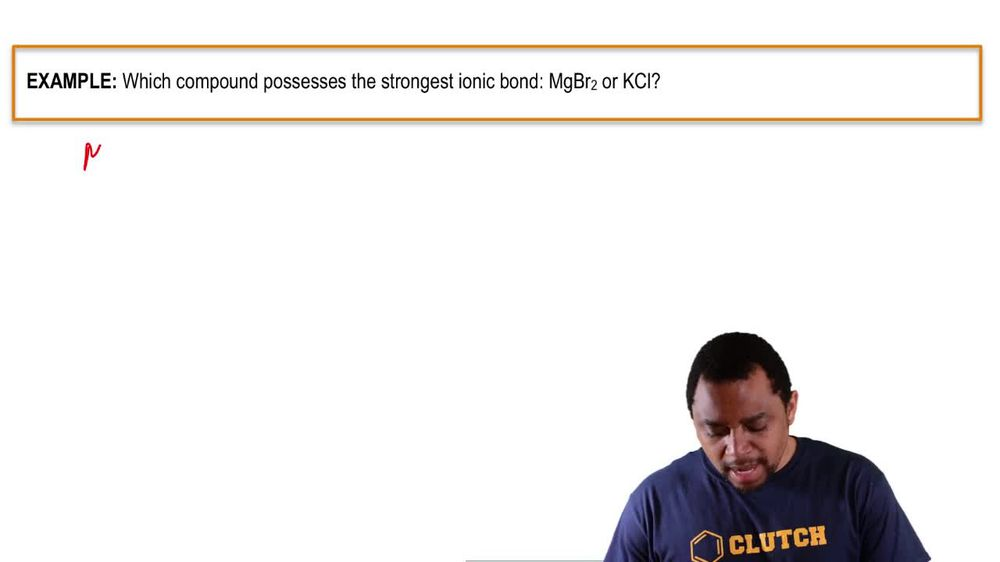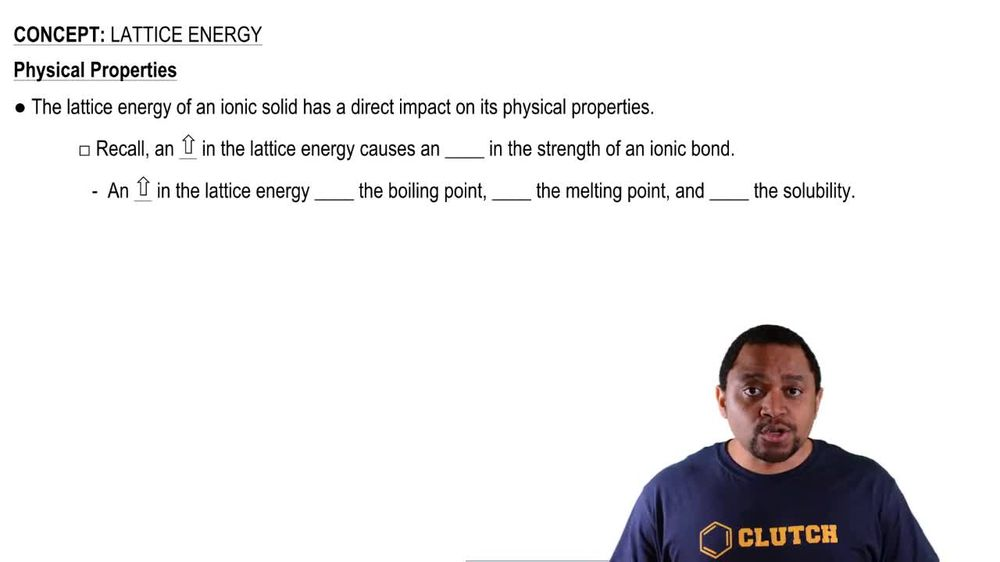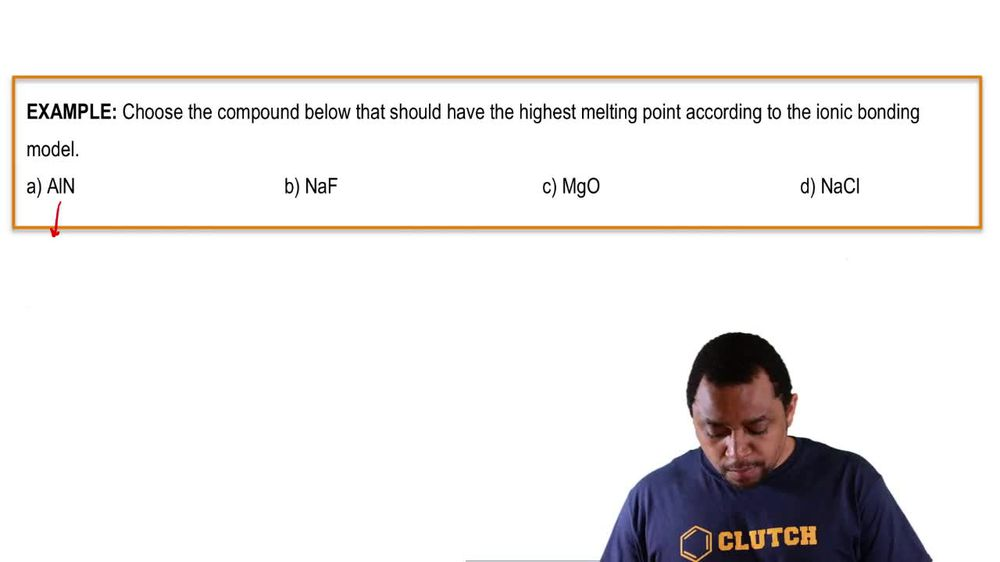Start typing, then use the up and down arrows to select an option from the list.
1. 11. Bonding & Molecular Structure2. Lattice Energy# Lattice Energy Example 1

by Jules Bruno
184 views
1
0
So here it says the lattice formation of magnesium bromide is the energy change for which of the following processes. So remember, in a lattice formation reaction, we have the Ionic gasses combining to form an ionic solid. So here, magnesium is in group two way, so it's two plus boards charge. So this is good, bro means and groups seven a. So it's minus one. So this is good. And then remember they combined together to form an ionic solid, not a gas. So this is out, he answered. The only answer that can work is option three. None of the others work because they don't have ions to begin with that combined to form an ionic solids. So here are answer would be choice, see?